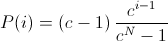io.jenetics

## Class ExponentialRankSelector<G extends Gene<?,G>,C extends Comparable<? super C>>

• All Implemented Interfaces:
Selector<G,C>

public final class ExponentialRankSelector<G extends Gene<?,G>,C extends Comparable<? super C>>
extends ProbabilitySelector<G,C>

An alternative to the "weak" LinearRankSelector is to assign survival probabilities to the sorted individuals using an exponential function.,

where c must within the range [0..1).

A small value of c increases the probability of the best phenotypes to be selected. If c is set to zero, the selection probability of the best phenotype is set to one. The selection probability of all other phenotypes is zero. A value near one equalizes the selection probabilities.

This selector sorts the population in descending order while calculating the selection probabilities.

Since:
1.0
Version:
4.0
Author:
Franz Wilhelmstötter

• ### Fields inherited from class io.jenetics.ProbabilitySelector

_reverter, _sorted, POPULATION_COMPARATOR
• ### Constructor Summary

Constructors
Constructor and Description
ExponentialRankSelector()
Create a new selector with default value of 0.975.
ExponentialRankSelector(double c)
Create a new exponential rank selector.
• ### Method Summary

All Methods
Modifier and Type Method and Description
boolean equals(Object obj)
int hashCode()
protected double[] probabilities(Seq<Phenotype<G,C>> population, int count)
This method sorts the population in descending order while calculating the selection probabilities.
String toString()
• ### Methods inherited from class io.jenetics.ProbabilitySelector

probabilities, select
• ### Methods inherited from class java.lang.Object

clone, finalize, getClass, notify, notifyAll, wait, wait, wait
• ### Constructor Detail

• #### ExponentialRankSelector

public ExponentialRankSelector(double c)
Create a new exponential rank selector.
Parameters:
c - the c value.
Throws:
IllegalArgumentException - if c is not within the range [0..1).
• #### ExponentialRankSelector

public ExponentialRankSelector()
Create a new selector with default value of 0.975.
• ### Method Detail

• #### probabilities

protected double[] probabilities(Seq<Phenotype<G,C>> population,
int count)
This method sorts the population in descending order while calculating the selection probabilities.
Specified by:
probabilities in class ProbabilitySelector<G extends Gene<?,G>,C extends Comparable<? super C>>
Parameters:
population - The unsorted population.
count - The number of phenotypes to select. This parameter is not needed for most implementations.
Returns:
Probability array. The returned probability array must have the length population.size() and must sum to one. The returned value is checked with assert(Math.abs(math.sum(probabilities) - 1.0) < 0.0001) in the base class.
• #### hashCode

public int hashCode()
Overrides:
hashCode in class Object
• #### equals

public boolean equals(Object obj)
Overrides:
equals in class Object
• #### toString

public String toString()
Overrides:
toString in class Object

© 2007-2018 Franz Wilhelmstötter  (2018-02-14 08:27)